Courses

# Paper Folding Quiz II, Non Verbal Reasoning

## 15 Questions MCQ Test Non Verbal Reasoning | Paper Folding Quiz II, Non Verbal Reasoning

Description
This mock test of Paper Folding Quiz II, Non Verbal Reasoning for LR helps you for every LR entrance exam. This contains 15 Multiple Choice Questions for LR Paper Folding Quiz II, Non Verbal Reasoning (mcq) to study with solutions a complete question bank. The solved questions answers in this Paper Folding Quiz II, Non Verbal Reasoning quiz give you a good mix of easy questions and tough questions. LR students definitely take this Paper Folding Quiz II, Non Verbal Reasoning exercise for a better result in the exam. You can find other Paper Folding Quiz II, Non Verbal Reasoning extra questions, long questions & short questions for LR on EduRev as well by searching above.
QUESTION: 1

Solution:
QUESTION: 2

Solution:
QUESTION: 3

### Directions to Solve In each of the following problems, a square transparent sheet (X) with a pattern is given. Figure out from amongst the four alternatives as to how the patter would appear when the transparent sheet is folded at the dotted line. Question - Find out from amongst the four alternatives as to how the pattern would appear when the transparent sheet is folded at the dotted line.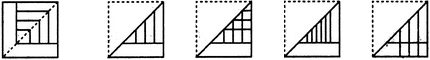(X)                     (1)                 (2)                 (3)                 (4)

Solution:
QUESTION: 4

In each of the following problems, a square transparent sheet (X) with a pattern is given. Figure out from amongst the four alternatives as to how the patter would appear when the transparent sheet is folded at the dotted line.

Question -

Find out from amongst the four alternatives as to how the pattern would appear when the transparent sheet is folded at the dotted line.(X)                     (1)                 (2)                 (3)                 (4)
Solution:
QUESTION: 5

In each of the following problems, a square transparent sheet (X) with a pattern is given. Figure out from amongst the four alternatives as to how the patter would appear when the transparent sheet is folded at the dotted line.

Question -

Find out from amongst the four alternatives as to how the pattern would appear when the transparent sheet is folded at the dotted line.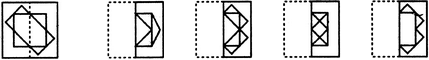(X)                     (1)                 (2)                 (3)                 (4)
Solution:
QUESTION: 6

In each of the following problems, a square transparent sheet (X) with a pattern is given. Figure out from amongst the four alternatives as to how the patter would appear when the transparent sheet is folded at the dotted line.

Question -

Find out from amongst the four alternatives as to how the pattern would appear when the transparent sheet is folded at the dotted line.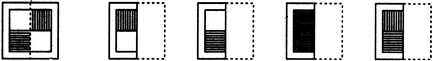(X)                     (1)                 (2)                 (3)                 (4)
Solution:
QUESTION: 7

In each of the following problems, a square transparent sheet (X) with a pattern is given. Figure out from amongst the four alternatives as to how the patter would appear when the transparent sheet is folded at the dotted line.

Question -

Find out from amongst the four alternatives as to how the pattern would appear when the transparent sheet is folded at the dotted line.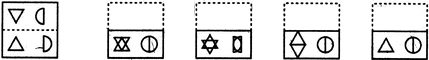(X)                     (1)                 (2)                 (3)                 (4)
Solution:
QUESTION: 8

Directions to Solve

In each of the following problems, a square transparent sheet (X) with a pattern is given. Figure out from amongst the four alternatives as to how the patter would appear when the transparent sheet is folded at the dotted line.

Question -

Find out from amongst the four alternatives as to how the pattern would appear when the transparent sheet is folded at the dotted line.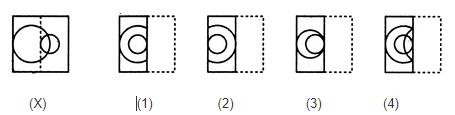Solution:
QUESTION: 9

In each of the following problems, a square transparent sheet (X) with a pattern is given. Figure out from amongst the four alternatives as to how the patter would appear when the transparent sheet is folded at the dotted line.

Question -

Find out from amongst the four alternatives as to how the pattern would appear when the transparent sheet is folded at the dotted line.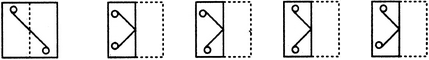(X)                     (1)                 (2)                 (3)                 (4)
Solution:
QUESTION: 10

In each of the following problems, a square transparent sheet (X) with a pattern is given. Figure out from amongst the four alternatives as to how the patter would appear when the transparent sheet is folded at the dotted line.

Question -

Find out from amongst the four alternatives as to how the pattern would appear when the transparent sheet is folded at the dotted line.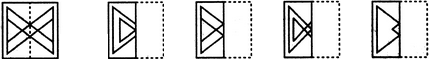(X)                     (1)                 (2)                 (3)                 (4)
Solution:
QUESTION: 11

In each of the following problems, a square transparent sheet (X) with a pattern is given. Figure out from amongst the four alternatives as to how the patter would appear when the transparent sheet is folded at the dotted line.

Question -

Find out from amongst the four alternatives as to how the pattern would appear when the transparent sheet is folded at the dotted line.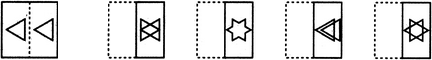(X)                     (1)                 (2)                 (3)                 (4)
Solution:
QUESTION: 12

In each of the following problems, a square transparent sheet (X) with a pattern is given. Figure out from amongst the four alternatives as to how the patter would appear when the transparent sheet is folded at the dotted line.

Question -

Find out from amongst the four alternatives as to how the pattern would appear when the transparent sheet is folded at the dotted line.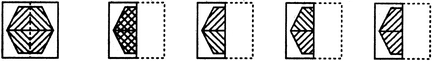(X)                     (1)                 (2)                 (3)                 (4)
Solution:
QUESTION: 13

In each of the following problems, a square transparent sheet (X) with a pattern is given. Figure out from amongst the four alternatives as to how the patter would appear when the transparent sheet is folded at the dotted line.

Question -

Find out from amongst the four alternatives as to how the pattern would appear when the transparent sheet is folded at the dotted line.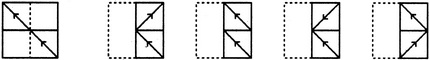(X)                     (1)                 (2)                 (3)                 (4)
Solution:
QUESTION: 14

In each of the following problems, a square transparent sheet (X) with a pattern is given. Figure out from amongst the four alternatives as to how the patter would appear when the transparent sheet is folded at the dotted line.

Question -

Find out from amongst the four alternatives as to how the pattern would appear when the transparent sheet is folded at the dotted line.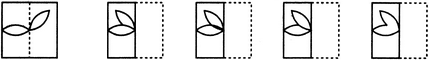(X)                     (1)                 (2)                 (3)                 (4)
Solution:
QUESTION: 15

In each of the following problems, a square transparent sheet (X) with a pattern is given. Figure out from amongst the four alternatives as to how the patter would appear when the transparent sheet is folded at the dotted line.

Question -

Find out from amongst the four alternatives as to how the pattern would appear when the transparent sheet is folded at the dotted line.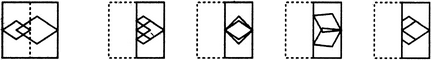(X)                     (1)                 (2)                 (3)                 (4)
Solution: